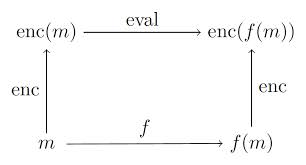## Introduction

A homomorphism is a structure-preserving map from one algebraic structure to another; see Wikipedia. Privacy experts are interested in homomorphic computation because it offers a way to perform computations on encrypted data, either in a distributed setting or in the cloud, thereby handling many of the headaches associated with storing/moving/anonymizing data. Homomorphic computation also finds application in secure voting, verifyable computing etc.

A homomorphic encryption scheme is one that provides such a homomorphism along with the infrastructure to carry out the computations. The schemes provide algorithms for generating public and private keys. The public key is distributed to anyone and the private key is only needed by the one who does the decryption. There are several such schemes documented in Wikipedia. The main thing to know is that there are two flavours: partial and full. Partial schemes preserve structure for certain specified operations, say addition only, whereas full schemes do over all the standard arithmetic operations. The operations mentioned here are all in the context of the algebraic structure underneath, which for our purposes will be modular arithmetic where the modulus is some large number.

The current package homomorpheR implements the Paillier system which is a partially homomorphic; it provides an additive homomorphism. The implementation here borrows much from the Javascript implementation for a proof-of-concept system. Therefore, it is not yet ready for serious work. So there, you’ve been warned.

If you are interested in the mathematical details of the Paillier crytosystem, the main reference is (Paillier 1999). Volkhausen (2006) provides a detailed mathematical introduction; Michael O’Keefe (2008) is a gentler one. A simple application of the Paillier system for secure vote tallying is in (Choinyammbu 2009).

A bit of notation helps. Let $$x$$ be any message; for us, it is just a large integer. Denote $$E(x)$$ as the encrypted message and $$D(x)$$ as the decryption of $$x$$ in some scheme. If the scheme is homomorphic over addition, then we have $E(x) + E(y) = E(x + y)$

This means that calculating $$x+y$$ can be done by decrypting the sum of the encrypted values of $$x$$ and $$y$$. Thus, entities can merely exchange encrypted values throughout. This is pictorially shown in the figure (source: http://jeremykun.com/tag/homomorphic-encryption ) below:Homomorphic Computation

## Facilities

As a first step, a public and private key pair needs to be generated. In generating bits for cryptosystems, a secure random number source is needed; homomorpheR makes use of the R package sodium by Jeroen Ooms (based on Daniel J. Bernstein’s generators) in addition to gmp for arbitrary precision arithmetic.

library(homomorpheR)
keyPair <- PaillierKeyPair$new(modulusBits = 1024) Examine the keyPair object: keyPair ## <PaillierKeyPair> ## Public: ## clone: function (deep = FALSE) ## getPrivateKey: function () ## initialize: function (modulusBits) ## pubkey: PaillierPublicKey, R6 ## Private: ## privkey: PaillierPrivateKey, R6 The pubkey field can be distributed all interested parties but the private key, obtainable by getPrivateKey() should be kept secret for decryption. The main functions are the encrypt function for the public key, and the decrypt function for the private key. ## Some tests We can now perform some simple tests. But before that a simple function that encrypts and decrypts. encryptAndDecrypt <- function(x) keyPair$getPrivateKey()$decrypt(keyPair$pubkey\$encrypt(x))

Now we can encrypt and decrypt some numbers.

a <- gmp::as.bigz(1273849)
identical(a + 10, encryptAndDecrypt(a + 10))
##  TRUE

Now with a large set of numbers. The function random.bigz returns large random numbers.

m <- lapply(1:100, function(x) random.bigz(nBits = 512))
md <- lapply(m, encryptAndDecrypt)
identical(m, md)
##  TRUE